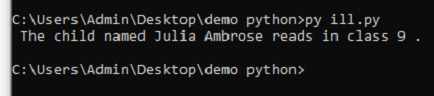# String Formatting Operator

#### String Operator

The String is one of the most used data types in Python. If we define a String within a single quote or in the double quote, python treats the String the same way.

```E.g., var1 = [‘Hello’, ‘World’]
var2 = [“Hello”, “World”]
Result:- var1 = var2.

String uses operators to manipulate its value or results. Python also provides operators to manipulate the value of the Strings.```
• “ + ” - Use to concatenate the two String.
• “ * ” - Use to multiply the value of the String.
• “ [] ” - Slices the String and returns the character written in the index.
• “ [ : ] ” - Returns the characters of the String in the given range.
• “ in ” - Returns boolean value for the character exists or not in the String.
• “ not in ” - Returns boolean value for the character nonexistence or existence in the String.
• “ r/R ” - Prints a raw string.
• “ % ” - Format String Operator

#### String Format Operator:

Python is known to be the most extraordinary language; it is because of these kinds of features. In Python, %, String format operator is the same as we have seen earlier in C language. % creates the family of the data types.

#### Symbols and their data types are as follow in Python-

• %c = character
• %s = String conversion
• %i, %d = decimal integers
• %u = unsigned decimal integers
• %o = octal integer
• %x = hexadecimal integer
• %X = hexadecimal integer (Uppercase)
• %e = exponential expressions or notations
• %E = exponential expressions or notations (Uppercase)
• %f = real numbers floating point
• %G = shorter between %f and %E

#### Example Code:

#printing this child named Juila Ambrose reads in class 9 name = "Julia Ambrose" cl=9 print(" The child named %s reads in class %d ." % (name, cl))

#### OUTPUT#### Example Code:

# printing The list of absentees: [49, 20, 31] li = [49,20,31] print("The list of absentees : %s" % li)

#### OUTPUT